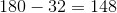MAP 4th Grade Math : Measurement and Data

Example Questions

Example Question #1 : Measurement And Data

What is the length of a rectangular room with a perimeter of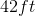and a width of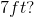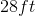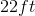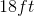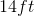Explanation: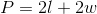We have the perimeter and the width, so we can plug those values into our equation and solve for our unknown.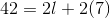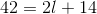Subtractfrom both sides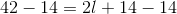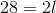Divideby both sides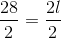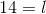Example Question #2 : Measurement And Data

David wants to put a fence around his backyard. How much fencing will he need if his backyard is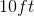by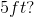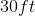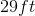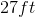Explanation:

The fence is going around the backyard, so this is a perimeter problem.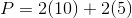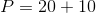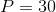Example Question #3 : Measurement And Data

If we wanted to make this angle into a straight line, how many more degrees would we need to add?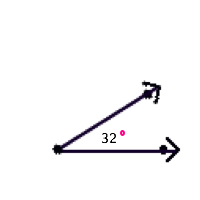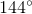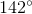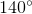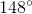A straight line has a measurement of. We can subtract to find how much more we'd need to have a straight line.